Home | | Business Maths 12th Std | Choose the Correct answer

## Chapter: 12th Business Maths and Statistics : Chapter 4 : Differential Equations

Maths: Differential Equations: Multiple choice questions with Answers, Solution - Book back 1 mark questions and answers with solution for Exercise Problems

1. The degree of the differential equation(a) 1

(b) 2

(c) 3

(d) 4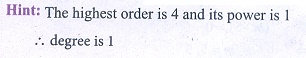2. The order and degree of the differential equation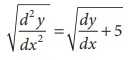are respectively

(a) 2 and 3

(b) 3 and 2

(c) 2 and 1

(d) 2 and 23. The order and degree of the differential equationare respectively.

(a) 2 and 6

(b) 3 and 6

(c) 1 and 4

(d) 2 and 4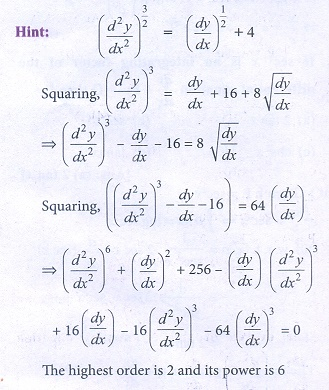4. The differential equationis

(a) of order 2 and degree 1

(b) of order 1 and degree 3

(c) of order 1 and degree 6

(d) of order 1 and degree 25. The differential equation formed by eliminating a and b from y = aex + be−x isAns: (a)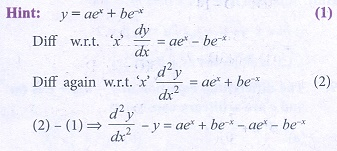6. If y = cx + c − c3 then its differential equation isAns: (a)7. The integrating factor of the differential equation dx/dy + Px = Q is

(a) e∫ Pdx

(b) e∫ Pdx

(c) ∫ Pdy

(d) e∫ Pdy8. The complementary function of ( D2 + 4) y = e2x is

(a) ( Ax + B )e2x

(b) ( Ax + B )e−2x

(c) A cos 2x + B sin 2x

(d) Ae−2 x + Be2x9. The differential equation of y = mx + c is (m and c are arbitrary constants)

(a) d2y / dx2 = o

(b) y = x dy/dx + c

(c) xdy + ydx = 0

(d) ydx − xdy = 010. The particular integral of the differential equation is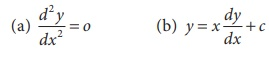(c) x2e4x

(d) xe4x11. Solution of dx/dy + Px = 0

(a) x = cepy

(b) x = ce− py

(c) x = py + c

(d) x = cy12. If sec2x is an integrating factor of the differential equation dy/dx + Py = Q then P =

(a) 2tan x

(b) sec x

(c) cos2x

(d) tan2x13. The integrating factor of x dy/dx − y = x2 is

(a) -1/x

(b) 1/x

(c) log x

(d) x14. The solution of the differential equation dy/dx + Py = Q where P and Q are the function of x is dx

(a) y = ∫Qe∫ Pdxdx + c

(b) y = ∫Qe−∫ Pdxdx + c

(c) ye∫ Pdx = ∫Qe∫ Pdxdx + c

(d) ye∫ Pdx = ∫Qe−∫ Pdxdx + C

15. The differential equation formed by eliminating A and B from y = e−2x ( A cos x + B sin x) is

(a) y2 − 4 y1 + 5 = 0

(b) y2+ 4y – 5 = 0

(c) y2 − 4 y1 − 5 = 0

(d) y2 + 4 y1 + 5 = 0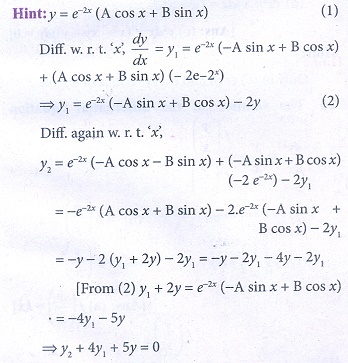16. The particular integral of the differential equation f ( D) y = eax where f ( D) = ( D  a)2

(a) x2/2 eax

(b) xeax

(c) x/2 eax

(d) x2eax17. The differential equation of x2 + y2 = a2

(a) xdy+ydx=0

(b) ydx–xdy=0

(c) xdx–ydx=0

(d) xdx+ydy=018. The complementary function of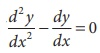is

(a) A + Bex

(b) ( A + B)ex

(c) ( Ax + B )ex

(d) Aex + B19. The P.I of (3D2 + D − 14)y = 13e2x is

(a) x/2 e2x

(b) xe2x

(c) x2/2 e2x

(d) 13xe2x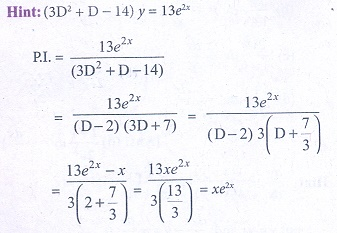20. The general solution of the differential equation dy/dx = cos x is

(a) y = sin x + 1

(b) y = sin x - 2

(c) y = cos x + c , c is an arbitrary constant

(d) y = sin x + c, c is an arbitrary constant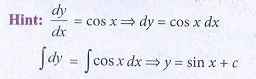21. A homogeneous differential equation of the form dy/ dx = f (y/x)  can be solved by making substitution,

(a) y = v x

(b) v = y x

(c) x = v y

(d) x = v

22. A homogeneous differential equation of the form dx/ dy = f (x/y)  can be solved by making substitution,

(a) x = v y

(b) y = v x

(c) y = v

(d) x = v

23. The variable separable form ofby taking y = vx andisAns: (d)24. Which of the following is the homogeneous differential equation?

(a) ( 3x − 5) dx = ( 4 y − 1) dy

(b) xydx - (xy3) dy=0

(c) y2 dx + ( x2 − xy − y2 ) dy = 0

(d) (x2 + y) dx = (y2 + x)dy25. The solution of the differential equationis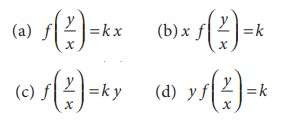Ans: (a)Tags : Differential Equations , 12th Business Maths and Statistics : Chapter 4 : Differential Equations
Study Material, Lecturing Notes, Assignment, Reference, Wiki description explanation, brief detail
12th Business Maths and Statistics : Chapter 4 : Differential Equations : Choose the Correct answer | Differential Equations